# Set of prime divisors of values of nonconstant polynomial with integer coefficients is infinite

## Statement

Suppose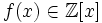is a nonconstant polynomial. Consider the set: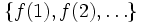.

Then, there are infinitely many primessuch thatdividesfor some natural number.

## Proof

Given: A nonconstant polynomial.

To prove: The set of prime divisors of,varying over positive integers, is infinite.

Proof:

1. We can assume thatis primitive and irreducible over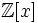. In particular, we can assume that the gcd of the coefficients ofis, and thathas a nonzero constant term: Ifdoesn't already satisfy these conditions, pick an irreducible primitive factor polynomial of.
2. Suppose there are only finitely many primesfor which we can find asuch thatdivides. Letbe these primes. Let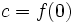and letbe any natural number. Consider. This can be written astimes a polynomial in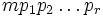, with the latter polynomial having constant term. In particular, the latter term cannot be a multiple of any, and hence must be. However, this cannot be true for every natural numberunlessis a constant polynomial.